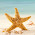## document.write(ssyby);

### Fun simulating Wimbledon in R and Python

R and Python have different strengths. There's little you can do in R you absolutely can't do in Python and vice versa, but there's a lot of stuff that's really annoying in one and nice and simple in the other. I'm sure simulations can be run in R, but it seems frightfully tricky. Recently I wrote a simple Tennis simulator in Python, which copies all the Tennis rules, and allows player skill to be entered. It would print running scores as the game went, or if asked to, would run a large number of matches and calculate win percentages. I quickly found that the structure of Tennis is such that marginal gains are really valuable, as only a small increase in skill translated into a large increase in number of matches won. How about mapping this? what does the relationship between skill and tennis matches won look like? Where exactly is the cut-off point of skill, below which winning is not just lucky, but impossible? Does increasing the 'serve bonus', meaning service holds are very likely, improve or reduce the odds for the underdog?

To answer these questions I decided to run the Python simulator from within R, and collect the output for simulations under different conditions. The first step was to get the Python script running through R, which meant making it executable. The simulator I used is the one I posted here previously. To this I only added the following code to make it run in the command line. All this does is take the arguments from the command prompt and map them to variables, which Python then send to runApp simulator:

``````def main(argv=None):
if argv is None:
argv =sys.argv

if not argv[1:]:
sys.exit()
number=int(argv)
player1=argv
skill1=int(argv)
player2=argv
skill2=int(argv)
serveBonus=float(argv)
runApp(number,player1,skill1,player2,skill2,serveBonus)

if __name__ == "__main__":
sys.exit(main())
``````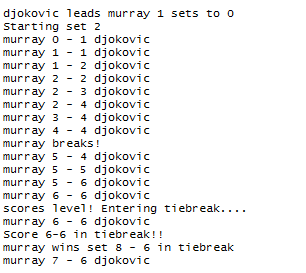My output from the simulator
Having done this we can call the simulator from within R easily enough.

Navigate to the directory with the tennis simulator, and launch this: system(paste0(“python tennis.py 1 Murray 90 Djokovic 100 0.5” )) In my case Murray ended up winning a thrilling match 4-6, 6-3, 7-5, 6-4, despite Djokovic being the odds on favourite.

Now what we want to do is capture the simulator output in R. To do this I set the simulator to play each match 100 times, and return only the number of times player1 won. R let's you add a 'intern=T' argument to the system call, meaning R will capture whatever shows on screen after the Python script has run. Leveraging this, we can set R to loop through different skill levels, collect the number of victories at each, and plot them. This is not an efficient approach, it could be done better within Python or with a single call, but for simplicity's sake, we will proceed as follows:

``````results = NULL
minSkill = 0
maxSkill = 200
for (i in minSkill:maxSkill) {
results = c(results, as.numeric(system(paste0("python tennis.py 100 murray ",
i, " djokovic 100 0.5"), intern = T)))
}
``````

the code above sets a minimum and a maximum skill level, then the loop launches the python script the appropriate number of times, substituting Murray's skill level for “i”, in this case starting at 0 and all the way up to 200
We collect each output in 'results'. Let's add those to a table, with the corresponding skill-level in a separate column, and plot this with ggplot:

``````library(ggplot2)
df=data.frame(results)
df\$skill=minSkill:maxSkill
ggplot(df,aes(skill,results))+
geom_hline(yintercept=50,colour="red")+ #add the reference point of 50% matches won
geom_point()+ #show individual points
geom_smooth(span=.5)+ #trend line
ylab("n matches won (of 100)")+
ggtitle("Number of matches won by Murray v Djokovic (skill=100)")``````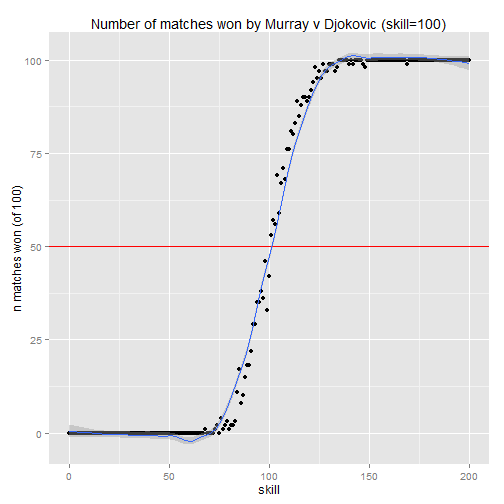Apparently unless Murray is at least 70% as good as Djokovic, he cannot win. So here is a clear illustration of how the structure of numerous points adding into games into sets acts to ensure the best player has a high chance of winning.
Now let's say for the sake of argument that Murray and Djokovic are evenly matched, i.e. both have a skill-level of 100, but thanks to the home support Murray ups his game by 5 percent. How does that affect his odds of victory? Let's zoom in on the chart:

``````library(ggplot2)
ggplot(df[df\$skill>90&df\$skill<110,],aes(skill,results))+
geom_hline(yintercept=50,colour="red")+ #add the reference point of 50% matches won
geom_point()+ #show individual points
geom_smooth(method="lm")+ #trend line
ylab("n matches won (of 100)")+
ggtitle("Number of matches won by Murray v Djokovic (skill=100)")
``````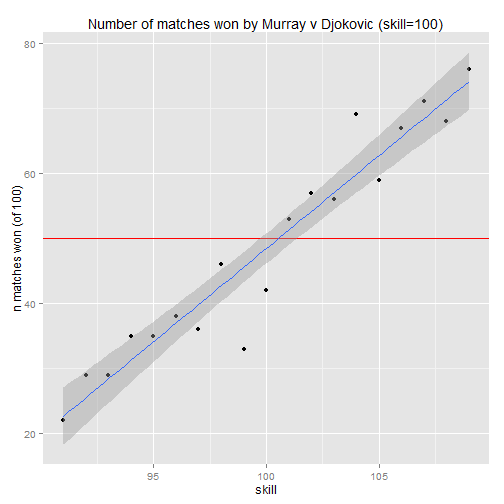The trend-line doesn't go precisely through the predicted 50% mark, but by extrapolating it looks as if a 5% improvement in skill increases the odds of victory from 50% to 65%. In other words, at the elite level, where margins are extremely small, marginal gains are huge in tennis - roughly 3% increase in victory odds for 1% increase in skill.
Now, what about playing only two sets?

``````results1=NULL
minSkill=30
maxSkill=180
for(i in minSkill:maxSkill){
results1=c(results1,as.numeric(system(paste0("python tennis.py 100 murray ",i," djokovic 100 0" ),intern=T)))
}
results2=NULL
minSkill=30
maxSkill=180
for(i in minSkill:maxSkill){
results2=c(results2,as.numeric(system(paste0("python tennis2.py 100 murray ",i," djokovic 100 2" ),intern=T)))
}

df1=data.frame(results1)
df1\$skill=minSkill:maxSkill
df1\$sets="three"
colnames(df1) <- span=""> "results"
df2=data.frame(results2)
df2\$skill=minSkill:maxSkill
df2\$sets="two"
colnames(df2) <- span=""> "results"
df=rbind(df1,df2)

ggplot(df[df\$skill>50&df\$skill<150,],aes(skill,results,group=sets,colour=sets))+
geom_hline(yintercept=50,colour="red")+ #add the reference point of 50% matches won
geom_point()+ #show individual points
geom_smooth()+ #trend line
ylab("n matches won (of 100)")+
ggtitle("Number of matches won by Murray v Djokovic (skill=100)")``````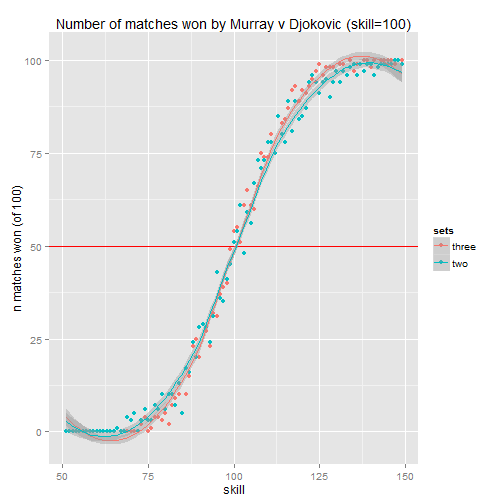Clearly playing three sets favours the stronger player. In a two set match the increased chance of winning due to an increase in skill is lower. Often the women's side is seen as weaker than the men's side, due to more surprises and new names making late stages of tournaments. However, at least part of the reason for this must be that luck plays a noticably larger role for two set matches than for three set matches.

1.2.